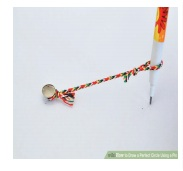Home | | Maths 7th Std | Circle

# Circle

In our daily life, we come across circular shapes in various places. For understanding of circular shapes, first let us see how to trace a circle through an activity.

Circle

In our daily life, we come across circular shapes in various places. For understanding of circular shapes, first let us see how to trace a circle through an activity.

Activity

Put a pin on a board, put a loop of string around it, and insert a pencil into the loop. Keep the string stretched and draw with the pencil. The pencil traces out a circle.What happens if we change the position of the pin? Do we get the same circle or a different circle? Can we make the string longer? Do we get a circle of the same size? Can we change the pencil to a pen? What happens to the circle? Yes, changing the pen to pencil or sketch pen will only change the colour of the circle but changing the position of the pin and length of the string will change the place where the circle is drawn and also the size of the circle. These two quantities namely, the position of the pin and the length of the string are important to specify the circle.

The position of the pin on the board is the centre (O) of the circle. The length of the string is the radius (r) of the circle.

While tracing a circle, the two positions of the string which falls on a straight line is the diameter (d) of the circle. It is twice the radius (d = 2r).

Try these

1. A few real life examples of circular shapes are given below.Give three more examples.

Bangle, Bicycle wheel and Clock.

2. Find the diameter of your bicycle wheel?

The diameter of my bicycle wheel is 70 cm.

3. If the diameter of the circle is 14cm, what will be it’s radius?

The diameter of the circle = 14 cm

radius = 14/2 = 7 cm

4. If the radius of a bangle is 2 inches then find the diameter.

The radius of a bangle = 2 inches

diameter = 2 × 2 = 4inches

Activity

Calculating the perimeter of a circle:

Make the students to draw five circles with different radii on a paper and instruct them to measure the radius, diameter and circumference of each of the circle using thread and scale. Note down the measurements in the following table.What do you infer from the above table? Can you conclude that the circumference of a circle is always greater than three times its diameter?

Tags : Measurements | Term 2 Chapter 2 | 7th Maths , 7th Maths : Term 2 Unit 2 : Measurements
Study Material, Lecturing Notes, Assignment, Reference, Wiki description explanation, brief detail
7th Maths : Term 2 Unit 2 : Measurements : Circle | Measurements | Term 2 Chapter 2 | 7th Maths